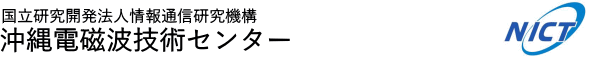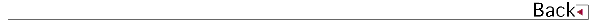# 2010\$BG/EY(B (2010.04\$B!A(B2011.03)

## \$B8&5fO@J8(B

• S. Nozawa,Y. Ogawa,S. Oyama,H. Fujiwara,T. Tsuda,A. Brekke,C. M. Hall,\$BB<;3(B \$BBY7<(B,\$B@nB<(B \$B@?<#(B,H. Miyaoka,R. Fujii,Tidal waves in the polar lower thermosphere observed using the EISCAT long run data set obtained in September 2005,JOURNAL OF GEOPHYSICAL RESEARCH,vol115

## \$B<}O?O@J8(B

• \$B@nB<(B \$B@?<#(B,\$B2VEZ(B \$B90(B,\$B?yC+(B \$BLPIW(B,\$BCf@n(B \$B>!9-(B,\$B%5%\$%I%m!<%VO3\$l9~\$_\$rDc8:\$5\$;\$??77?%P%\$%9%?%F%#%C%/!&%I%C%W%i!<4QB,%7%9%F%`(B,A NEW BISTATIC DOPPLER MEASUREMENT SYSTEM WITH REDUCED CONTAMINATION BY SIDELOBE ECHOES,IGARSS 2010
• \$B@nB<(B \$B@?<#(B,\$B?yC+(B \$BLPIW(B,\$B2VEZ(B \$B90(B,\$BCf@n(B \$B>!9-(B,\$B2-FlJPGH9_1+%l!<%@(BCOBRA\$B\$K\$h\$k2~NI7?%P%\$%9%?%F%#%C%/4QB,%7%9%F%`(B,\$BEE;R>pJsDL?.3X2q(B \$B1'Ch!&9R9T%(%l%/%H%m%K%/%98&5f2q(B
• \$BCf@n(B \$B>!9-(B,\$B2VEZ(B \$B90(B,\$B@nB<(B \$B@?<#(B,\$B9b66(B \$BD*9((B,\$B0f8}(B \$B=SIW(B,GROUND VALIDATION OF TRMM/PR MEASUREMENTS,ISPRS 2010

• \$B2VEZ(B \$B90(B,\$BCf@n(B \$B>!9-(B,\$B9b66(B \$BD*9((B,\$B0f8}(B \$B=SIW(B,\$B>.Eh@590(B,\$B8E@n6U;J(B,\$B;01:7r;K(B,\$BI4B+BY=S(B,\$B@P@Z9'G7(B,\$B@6?e<};J(B,\$B1|B<e9;@5J}K!\$N8!F\$(B,In-flight calibration plan for GPM /DPR,\$BEE;R>pJsDL?.3X2q!!1'Ch!&9R9T%(%l%/%H%m%K%/%98&5f2q(B

## \$B0lHL8}F,H/I=(B

• \$B5HB<(B \$BNh;R(B,\$B4];3(B \$BN4(B,\$B@nB<(B \$B@?<#(B,Reconstruction of topside ionospheric profile applying dynamic equilibrium based on GPS-TEC and ionosonde measurements,\$BF|K\CO5eOG@12J3XO"9g(B(JGU)
• \$B?yC+(B \$BLPIW(B,\$B@nB<(B \$B@?<#(B,\$B2VEZ(B \$B90(B,\$BCf@n(B \$B>!9-(B,\$B2~NI7?%P%\$%9%?%F%#%C%/9_1+%l!<%@%7%9%F%`(B ?\$BZ]3X2q(B
• \$B@nB<(B \$B@?<#(B,\$B?yC+(B \$BLPIW(B,\$B2VEZ(B \$B90(B,\$BCf@n(B \$B>!9-(B,\$B2~NI7?%P%\$%9%?%F%#%C%/9_1+%l!<%@%7%9%F%`!!!c%7%_%e%l!<%7%g%s8!F\$!d(B,\$BF|K\5\$>]3X2q!!=U5(Bg2q(B
• \$B>.ED(B \$BN=;R(B,\$B4d0f(B \$B9(FA(B,\$BBgLn(B \$BM50l(B,\$B@nB<(B \$B@?<#(B,\$B4X_7(B \$B?.Li(B,\$B@P0f(B \$B>;7{(B,\$B?eC+(B \$B9LJ?(B,\$BB<;3(B \$BBY7<(B,\$BEl5~ET>.6b0f;T>e6u\$N6-3&AX9bEYF|JQ2=\$N?dDj(B,\$BF|K\5\$>]3X2q(B \$B=U5(Bg2q(B
• \$B4d0f(B \$B9(FA(B,\$B>.ED(B \$BN=;R(B,\$B4X_7(B \$B?.Li(B,\$B@P0f(B \$B>;7{(B,\$BBgLn(B \$BM50l(B,\$B@nB<(B \$B@?<#(B,\$BB<;3(B \$BBY7<(B,\$B:9J,5[<}!?%I%C%W%i!<%i%\$%@!<\$K\$h\$kET;T6-3&AX\$NIw\$*\$h\$SMpN.7WB,(B,\$BF|K\CO5eOG@12J3XO"9g(B2010\$BG/Bg2q(B
• \$B4d0f(B \$B9(FA(B,\$B>.ED(B \$BN=;R(B,\$B4X_7(B \$B?.Li(B,\$B@P0f(B \$B>;7{(B,\$B?eC+(B \$B9LJ?(B,\$BBgLn(B \$BM50l(B,\$B@nB<(B \$B@?<#(B,\$BB<;3(B \$BBY7<(B,\$B:9J,5[<}!?%I%C%W%i!<%i%\$%@!<\$K\$h\$k6-3&AXCf\$N1tD>N.E}7WNL\$N?dDj(B,\$BF|K\5\$>]3X2q(B2010\$BG/EY=U5(Bg2q(B
• \$B>.ED(B \$BN=;R(B,\$B4d0f(B \$B9(FA(B,\$B4X_7(B \$B?.Li(B,\$B@P0f(B \$B>;7{(B,\$BBgLn(B \$BM50l(B,\$B@nB<(B \$B@?<#(B,\$B?eC+(B \$B9LJ?(B,\$BB<;3(B \$BBY7<(B,\$BEl5~ET;T0h\$K\$*\$1\$k%j%b!<%H%;%s%5\$H%>%s%G\$rMQ\$\$\$?6-3&AX9bEYF|JQF0\$N4QB,(B,\$BCO5eEE<'5\$!&CO5eOG@17w3X2q(B \$B9gF1Bg2q(B
• \$B@nB<(B \$B@?<#(B,\$B?yC+(B \$BLPIW(B,\$B2VEZ(B \$B90(B,\$BCf@n(B \$B>!9-(B,\$B2-FlJPGH9_1+%l!<%@(BCOBRA\$B\$K\$h\$k2~NI7?%P%\$%9%?%F%#%C%/4QB,
• \$B@nB<(B \$B@?<#(B,2\$Bl\$N%j%"%k%?%\$%`%b%K%?\$rL\E*\$H\$7\$?2~NI7?%P%\$%9%?%F%#%C%/9_1+%l!<%@4QB,%7%9%F%`\$N8&5f3+H/(B,\$B;:3X41O"7H?d?J2q5D(B
• \$BCf@n(B \$B>!9-(B,\$B2VEZ(B \$B90(B,\$B0f8}(B \$B=SIW(B,Onboard Calibration Plan of GPM/DPR,4th GPM International GV Workshop
• \$B:4C](B \$B@?(B,\$B@nB<(B \$B@?<#(B,\$B2VEZ(B \$B90(B,\$B=P@\$\$f\$+\$j(B,\$BCf@n(B \$B>!9-(B,\$B0f8}(B \$B=SIW(B,OCEAN SURFACE BACKSCATTERING AT EXTREMELY LOW GRAZING ANGLES OBSERVED BY C-BAND POLARIMETRIC DOPPLER WEATHER RADAR: DOPPLER SPECTRUM ANALYSIS,2010 IEEE International Geoscience and Remote Sensing Symposium
• \$B2VEZ(B \$B90(B,\$B@nB<(B \$B@?<#(B,\$B?yC+(B \$BLPIW(B,\$BCf@n(B \$B>!9-(B,\$B9b66(B \$BD*9((B,\$B0f8}(B \$B=SIW(B,\$BA45e9_?e4QB,7W2h!J(BGPM\$B!Ke\$G\$N9;@5
• \$B@nB<(B \$B@?<#(B,\$B?yC+(B \$BLPIW(B,\$B2VEZ(B \$B90(B,\$BCf@n(B \$B>!9-(B,A New Bistatic Weather Radar System with Reduced Contamination by Sidelobe Echoes,International Symposium on the 25th Anniversary of the MU Radar
• N. Balan,\$B@nB<(B \$B@?<#(B,Y. Otsuka,M. Yamamoto,S. Fukao,New aspects of the ionosphere and thermosphere revealed by MU radar,International Symposium on the 25th Anniversary of the MU Radar
• \$BDTNb(B,\$B?yC+(B \$BLPIW(B,\$B;T@n9a(B,\$B;3FbC#Lp(B,\$B?9K\>2A(B,2010\$BG/=)5(3\$MN3X2q(B
• \$B;3FbC#Lp(B,\$B?yC+(B \$BLPIW(B,\$B;T@n9a(B,\$BDTNb(B,\$B?9K\>eN.0h\$K\$*\$1\$k?aAwN.\$HIwB.\$N4X78\$N5(@a0MB8@-(B,\$B3\$MN3X2q!!(B2010\$BG/=)5(Bg2q(B
• \$B2VEZ(B \$B90(B,\$B@nB<(B \$B@?<#(B,\$B?yC+(B \$BLPIW(B,\$BCf@n(B \$B>!9-(B,\$B9b66(B \$BD*9((B,\$B0f8}(B \$B=SIW(B,\$BA45e9_?e4QB,7W2h!J(BGPM\$B!Ke\$G\$N30It9;@5\$N\$?\$a\$N%l!<%@9;@54o3+H/(B,Development of Radar Calibrators for the Dual-frequency Precipitation Radar (DPR) installed on the Global Precipitation Measurement (GPM) primary satellite,Asia-Pacific Radio Science Conference (AP-RASC)\$B!!(B
• \$B@nB<(B \$B@?<#(B,\$B?yC+(B \$BLPIW(B,\$B2VEZ(B \$B90(B,\$BCf@n(B \$B>!9-(B,A New Bistatic Doppler Measurement System with Reduced Sidelobe Contamination,2010 Asia-Pacific Radio Science Conference
• T. Futatsuya,\$B@nB<(B \$B@?<#(B,T. Miyake,K. Ishisaka,Y. Ashihara,\$BB<;3(B \$BBY7<(B,I. Nagano,T. Okada,Study on the estimation of the electron density profile in the lower ionosphere with time domain Full wave analysis,2010 Asia-Pacific Radio Science Conference
• \$BCf@n(B \$B>!9-(B,\$B2VEZ(B \$B90(B,\$B@nB<(B \$B@?<#(B,\$B?yC+(B \$BLPIW(B,\$B0f8}(B \$B=SIW(B,Raindrop Size Distribution Measurements for GPM/DPR Algorithm Development,AOGS 2010
• \$B?yC+(B \$BLPIW(B,\$B@nB<(B \$B@?<#(B,\$BCf@n(B \$B>!9-(B,\$B2VEZ(B \$B90(B,GPM(\$BA45e9_?e4QB,7W2h(B)/DPR(\$BFs<~GH9_?e%l!<%@(B) \$B50F;>e9;@5MQ%l!<%@9;@54o\$N3+H/(B,\$BF|K\5\$>]3X2q!!=)5(Bg2q(B
• \$BCf@n(B \$B>!9-(B,\$B2VEZ(B \$B90(B,\$B@nB<(B \$B@?<#(B,\$B?yC+(B \$BLPIW(B,\$BLnCf(B \$BM}?-(B,GPM/DPR \$BCO>e8!>Z4QB,\$N\$?\$a\$N0\F0<0CO>e9_?e4QB,%7%9%F%`\$N3+H/(B,\$BF|K\5\$>]3X2q!!=)5(Bg2q(B
• \$B@nB<(B \$B@?<#(B,\$B?yC+(B \$BLPIW(B,\$B2VEZ(B \$B90(B,\$BCf@n(B \$B>!9-(B,\$B2-FlJPGH9_1+%l!<%@(BCOBRA\$B\$rMQ\$\$\$?2~NI7?%P%\$%9%?%F%#%C%/9_1+%l!<%@%7%9%F%`Z]3X2q!!=)5(Bg2q(B
• \$B:4F#(B \$B?82p(B,\$B2VEZ(B \$B90(B,\$B@nB<(B \$B@?<#(B,\$B?yC+(B \$BLPIW(B,\$B>eED(B \$BGn(B,\$B@P86(B \$B@5?N(B,\$B5\$>]D#6K:BI8%l!<%@!<%G!<%?\$N3hMQJ}K!(B -\$B2-Fl;e?t%l!<%@!<\$H(BCOBRA\$B\$N(Bdual-Doppler\$B4QB,(B-,\$BF|K\5\$>]3X2q(B2010\$BG/EY=)5(Bg2q(B
• \$B@nB<(B \$B@?<#(B,\$B?yC+(B \$BLPIW(B,\$B2VEZ(B \$B90(B,\$BCf@n(B \$B>!9-(B,\$B2-FlJPGH9_1+%l!<%@(BCOBRA\$B\$K\$h\$k2~NI7?%P%\$%9%?%F%#%C%/4QB,%7%9%F%`\$NZ
• \$BCf@n(B \$B>!9-(B,\$B2VEZ(B \$B90(B,\$B@nB<(B \$B@?<#(B,\$B?yC+(B \$BLPIW(B,Raindrop size distribution measurements for TRMM/PR and GPM/DPR algorithm development,NASA Precipitation Measurement Missions (PMM) Science Team Meeting
• \$B?9K\>
• \$B?yC+(B \$BLPIW(B,\$B2VEZ(B \$B90(B,\$B@nB<(B \$B@?<#(B,NICT\$B1s5wN%3\$MN%l!<%@M?Fa9q6I!&@P3@6I\$N:FMxMQ\$H5;=Q3+H/(B,\$B3\$MN%l!<%@8&5f=82q(B
• \$B;3FbC#Lp(B,\$B?yC+(B \$BLPIW(B,\$B;T@n9a(B,\$BDTNb(B,\$B?9K\>eN.0h\$K\$*\$1\$k?aAwN.\$HIwB.\$N4X78\$N0MB8@-(B,\$B3\$MN%l!<%@8&5f=82q(B
• \$B?yC+(B \$BLPIW(B,\$B2VEZ(B \$B90(B,\$B@nB<(B \$B@?<#(B,\$B2-FlK\Eg@>4_0h\$K\$*\$1\$kig5\$O0O"B34QB,(B,HyARC-NICT\$B8&5f=82q(B
• \$B@nB<(B \$B@?<#(B,\$B?yC+(B \$BLPIW(B,\$B2VEZ(B \$B90(B,\$BCf@n(B \$B>!9-(B,\$B%=%U%H%&%'%"L5@~\$rMQ\$\$\$?%P%\$%9%?%F%#%C%/
• \$B;T@n9a(B,\$B?yC+(B \$BLPIW(B,\$B;3FbC#Lp(B,\$B5H@nM5(B,\$BDTNb(B,\$B?9K\>
• \$B;T@n9a(B,\$B?yC+(B \$BLPIW(B,\$BDTNb(B,\$B;3FbC#Lp(B,\$B?9K\>
• \$B@nB<(B \$B@?<#(B,\$B?yC+(B \$BLPIW(B,\$B2VEZ(B \$B90(B,COBRA\$B\$NMnMkHo32\$K\$D\$\$\$F(B,HyARC-NICT\$B6&F18&5f=82q(B
• \$B:4F#(B \$B?82p(B,\$B2VEZ(B \$B90(B,\$B@nB<(B \$B@?<#(B,\$B?yC+(B \$BLPIW(B,\$BCf@n(B \$B>!9-(B,\$BB<;3(B \$BBY7<(B,\$B8EK\(B \$B=_0l(B,\$BDEED(B \$BIRN4(B,400MHz\$BBS(BWPR/RASS\$B\$K\$h\$kIwB.\$H5\$29%W%m%U%!%\$%k\$NDj>o4QB,\$N8=>u\$H2]Bj(B,NICT-HyARC \$BJ?@.(B22\$BG/EY6&F18&5f=82q(B

## 1990\$B!A(B1991\$B!d(B1992\$B!d(B1993\$B!d(B1994\$B!d(B1995\$B!d(B1996\$B!d(B1997\$B!d(B1998\$B!d(B 1999\$B!d(B2000\$B!d(B2001\$B!d(B2002\$B!d(B2003\$B!d(B2004\$B!d(B2005\$B!d(B2006\$B!d(B2007\$B!d(B 2008\$B!d(B2009\$B!d(B2010\$B!d(B2011\$B!d(B2012\$B!d(B2013\$B!d(B2014\$B!d(B2015\$B!d(B2016\$B!d(B2017